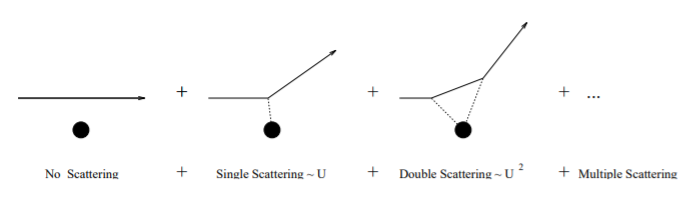# 13.1: Born Series, Green Functions - A Hint of Quantisation of the Field

$$\newcommand{\vecs}{\overset { \rightharpoonup} {\mathbf{#1}} }$$ $$\newcommand{\vecd}{\overset{-\!-\!\rightharpoonup}{\vphantom{a}\smash {#1}}}$$$$\newcommand{\id}{\mathrm{id}}$$ $$\newcommand{\Span}{\mathrm{span}}$$ $$\newcommand{\kernel}{\mathrm{null}\,}$$ $$\newcommand{\range}{\mathrm{range}\,}$$ $$\newcommand{\RealPart}{\mathrm{Re}}$$ $$\newcommand{\ImaginaryPart}{\mathrm{Im}}$$ $$\newcommand{\Argument}{\mathrm{Arg}}$$ $$\newcommand{\norm}{\| #1 \|}$$ $$\newcommand{\inner}{\langle #1, #2 \rangle}$$ $$\newcommand{\Span}{\mathrm{span}}$$ $$\newcommand{\id}{\mathrm{id}}$$ $$\newcommand{\Span}{\mathrm{span}}$$ $$\newcommand{\kernel}{\mathrm{null}\,}$$ $$\newcommand{\range}{\mathrm{range}\,}$$ $$\newcommand{\RealPart}{\mathrm{Re}}$$ $$\newcommand{\ImaginaryPart}{\mathrm{Im}}$$ $$\newcommand{\Argument}{\mathrm{Arg}}$$ $$\newcommand{\norm}{\| #1 \|}$$ $$\newcommand{\inner}{\langle #1, #2 \rangle}$$ $$\newcommand{\Span}{\mathrm{span}}$$$$\newcommand{\AA}{\unicode[.8,0]{x212B}}$$

Solving the Schrödinger equation using Green Functions automatically gives a solution in a form appropriate for scattering. By making the substitution $$E = \hbar^2 k^2/2\mu$$ and $$U(r) = (2\mu /\hbar^2 )V (r)$$ we can write the TISE as:

$[\nabla^2 + k^2 ] \Phi = U(r)\Phi \nonumber$

For $$U(r) = 0$$ this gives $$\phi_0(r) = Ae^{i{\bf k.r}}$$, a travelling wave. We now introduce a ‘Green’s Function’ for the operator $$[\nabla^2 + k^2 ]$$, which is the solution to the equation:

$[\nabla^2 + k^2 ]G(r) = \delta (r)G(r) \\ G(r) = − \text{ exp}(ikr)/4\pi r \nonumber$

$$\delta (r)$$ is the Dirac delta-function as is $$\delta (r)G(r)$$, since G(r) diverges at the origin. G(r) has the property that any function $$\Phi$$ which satisfies

$\Phi(r) = \phi_0 (r) +\int G \left(r-r^{\prime} \right) U \left(r^{\prime} \right) \Phi (r') d^{3} r^{\prime} \nonumber$

where $$\phi_0(r)$$ is the free particle solution, will be a solution to the TISE. Since $$\phi_0(r)$$ is the unscattered incoming wave, the second term must represent the scattered wave.

Thus the general solution to the TISE is given by:

$\Phi(r) = A e^{i k \cdot r}+\int G \left(r-r^{\prime} \right) U \left(r^{\prime} \right) \Phi (r') d^{3} r^{\prime} \nonumber$

In this expression, $$\Phi$$ appears on both sides. We can substitute for $$\Phi$$ using the same equation:

$\Phi(r) = A e^{i k \cdot r}+\int G \left(r-r^{\prime} \right) U \left(r^{\prime} \right) A e^{i k \cdot r^{\prime}} d^{3} r^{\prime}+\iint G \left(r-r^{\prime} \right) U \left(r^{\prime} \right) G \left(r^{\prime}-r^{\prime \prime} \right) U \left(r^{\prime \prime} \right) \Phi \left(r^{\prime \prime} \right) d^{3} r^{\prime} d^{3} r^{\prime \prime} \nonumber$

Repeated substitutions gives the Born series, terminated by a term involving $$\Phi (r)$$ itself. If the potential is weak, the higher order terms can be ignored. The first order term is just the matrix element between the incoming plane wave and the Green function: the Born approximation again! If we think of the potential $$U$$ as an operator, the first term represents the incoming wavefunction being operated on once. The second term represents the incoming wavefunction being operated on twice. And so forth. This suggests a way of quantising the effect of the field: The first order term corresponds to a single scattering event, the second order term to double scattering etc.Figure $$\PageIndex{1}$$: Born Series - scattering as series of terms

This page titled 13.1: Born Series, Green Functions - A Hint of Quantisation of the Field is shared under a CC BY 4.0 license and was authored, remixed, and/or curated by Graeme Ackland via source content that was edited to the style and standards of the LibreTexts platform; a detailed edit history is available upon request.